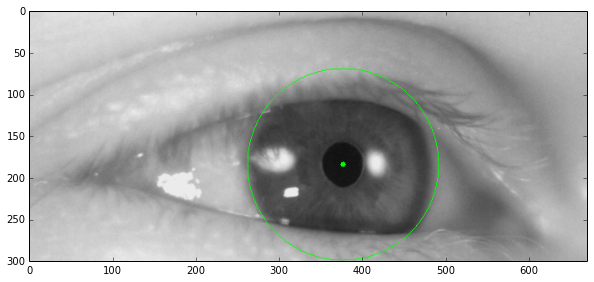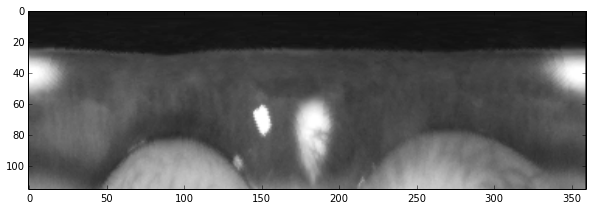Posted on

In the previous installments we've looked at Pupil Detection and Iris Detection. Now we'll look at unwrapping the image of the iris from a circular pattern to a rectangular one. We will use this later for some other algorithms.

So by applying the above mentioned algorithms we got two bits of information: the center of the pupil and the estimated radius of the iris. We can see them on the image below.In order to transform this circular iris into a rectangular image, we do the following:

``````import cv2
import numpy as np
import matplotlib.pyplot as plt

center = (376, 184)

nsamples = 360
samples = np.linspace(0, 2 * np.pi, nsamples)[:-1]

for theta in samples:
x = r * np.cos(theta) + center
y = r * np.sin(theta) + center

polar[r][theta * nsamples / 2.0 / np.pi] = image[y][x]

plt.figure(figsize=(10, 5))
plt.imshow(polar, cmap='gray')
``````

We create an empty image with resolution `iris_radius * 360` and then we iterate through this image and calculate the corresponding position in the circular image from the angle and the radius and copy this value to the new image.This post is also available as an iPython Notebook